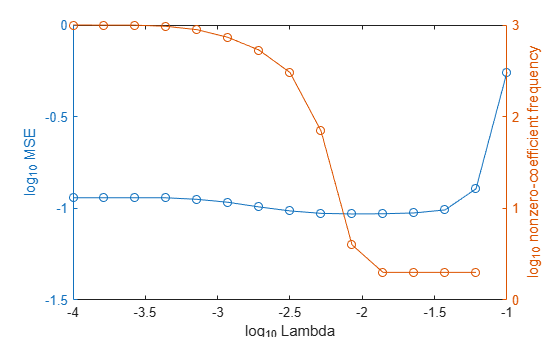Documentation

# selectModels

Class: RegressionLinear

Select fitted regularized linear regression models

## Syntax

``SubMdl = selectModels(Mdl,idx)``

## Description

example

````SubMdl = selectModels(Mdl,idx)` returns a subset of trained linear regression models from a set of linear regression models (`Mdl`) trained using various regularization strengths. The indices `idx` correspond to the regularization strengths in `Mdl.Lambda`, and specify which models to return.```

## Input Arguments

expand all

Linear regression models trained using various regularization strengths, specified as a `RegressionLinear` model object. You can create a `RegressionLinear` model object using `fitrlinear`.

Although `Mdl` is one model object, if `numel(Mdl.Lambda)` = L ≥ 2, then you can think of `Mdl` as L trained models.

Indices corresponding to regularization strengths, specified as a numeric vector of positive integers. Values of `idx` must be in the interval [1,L], where L = `numel(Mdl.Lambda)`.

Data Types: `double` | `single`

## Output Arguments

expand all

Subset of linear regression models trained using various regularization strengths, returned as a `RegressionLinear` model object.

## Examples

expand all

Simulate 10000 observations from this model

`$y={x}_{100}+2{x}_{200}+e.$`

• $X=\left\{{x}_{1},...,{x}_{1000}\right\}$ is a 10000-by-1000 sparse matrix with 10% nonzero standard normal elements.

• e is random normal error with mean 0 and standard deviation 0.3.

```rng(1) % For reproducibility n = 1e4; d = 1e3; nz = 0.1; X = sprandn(n,d,nz); Y = X(:,100) + 2*X(:,200) + 0.3*randn(n,1);```

Create a set of 15 logarithmically-spaced regularization strengths from $1{0}^{-4}$ through $1{0}^{-1}$.

`Lambda = logspace(-4,-1,15);`

Hold out 30% of the data for testing. Identify the test-sample indices.

```cvp = cvpartition(numel(Y),'Holdout',0.30); idxTest = test(cvp);```

Train a linear regression model using lasso penalties with the strengths in `Lambda`. Specify the regularization strengths, optimizing the objective function using SpaRSA, and the data partition. To increase execution speed, transpose the predictor data and specify that the observations are in columns.

```X = X'; CVMdl = fitrlinear(X,Y,'ObservationsIn','columns','Lambda',Lambda,... 'Solver','sparsa','Regularization','lasso','CVPartition',cvp); Mdl1 = CVMdl.Trained{1}; numel(Mdl1.Lambda)```
```ans = 15 ```

`Mdl1` is a `RegressionLinear` model. Because `Lambda` is a 15-dimensional vector of regularization strengths, you can think of `Mdl1` as 15 trained models, one for each regularization strength.

Estimate the test-sample mean squared error for each regularized model.

`mse = loss(Mdl1,X(:,idxTest),Y(idxTest),'ObservationsIn','columns');`

Higher values of `Lambda` lead to predictor variable sparsity, which is a good quality of a regression model. Retrain the model using the entire data set and all options used previously, except the data-partition specification. Determine the number of nonzero coefficients per model.

```Mdl = fitrlinear(X,Y,'ObservationsIn','columns','Lambda',Lambda,... 'Solver','sparsa','Regularization','lasso'); numNZCoeff = sum(Mdl.Beta~=0);```

In the same figure, plot the MSE and frequency of nonzero coefficients for each regularization strength. Plot all variables on the log scale.

```figure; [h,hL1,hL2] = plotyy(log10(Lambda),log10(mse),... log10(Lambda),log10(numNZCoeff)); hL1.Marker = 'o'; hL2.Marker = 'o'; ylabel(h(1),'log_{10} MSE') ylabel(h(2),'log_{10} nonzero-coefficient frequency') xlabel('log_{10} Lambda') hold off```Select the index or indices of `Lambda` that balance minimal classification error and predictor-variable sparsity (for example, `Lambda(11)`).

```idx = 11; MdlFinal = selectModels(Mdl,idx);```

`MdlFinal` is a trained `RegressionLinear` model object that uses `Lambda(11)` as a regularization strength.

## Tips

One way to build several predictive linear regression models is:

1. Hold out a portion of the data for testing.

2. Train a linear regression model using `fitrlinear`. Specify a grid of regularization strengths using the `'``Lambda``'` name-value pair argument and supply the training data. `fitrlinear` returns one `RegressionLinear` model object, but it contains a model for each regularization strength.

3. To determine the quality of each regularized model, pass the returned model object and the held-out data to, for example, `loss`.

4. Identify the indices (`idx`) of a satisfactory subset of regularized models, and then pass the returned model and the indices to `selectModels`. `selectModels` returns one `RegressionLinear` model object, but it contains `numel(idx)` regularized models.

5. To predict class labels for new data, pass the data and the subset of regularized models to `predict`.